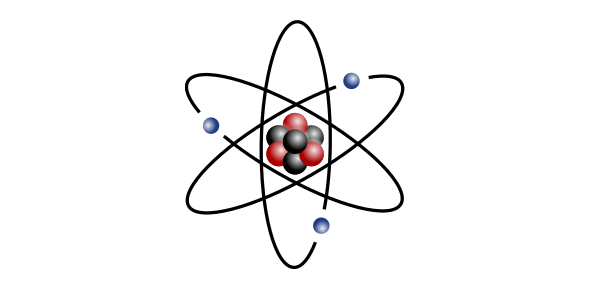Atom Basics Quiz

10 Questions | Total Attempts: 12372SettingsWhen we look at the scientific field of chemistry, there is arguably no more important topic that the atom. Once you start studying it, however, the subject matter can seem tough to come to grips with. Today, we aim to help you in learning the basics of the topic. Let’s see how much you know!

• 1.
The three basic components of an atom are:
• A.

Protons, neutrons, and ions

• B.

Protons, neutrons, and electrons

• C.

Protons, neutrinos, and ions

• D.

Protium, deuterium, and tritium

• 2.
An element is determined by the number of:
• A.

Atoms

• B.

Electrons

• C.

Neutrons

• D.

Protons

• 3.
The nucleus of an atom consists of:
• A.

Electrons

• B.

Neutrons

• C.

Protons and neutrons

• D.

Protons, neutrons, and electrons

• 4.
A single proton has what electrical charge?
• A.

No charge

• B.

Positive charge

• C.

Negative charge

• D.

Either a positive or negative charge

• 5.
Which particles have approximately the same size and mass as each other?
• A.

Neutrons and electrons

• B.

Electrons and protons

• C.

Protons and neutrons

• D.

None - they are all very different in size and mass

• 6.
Which two particles would be attracted to each other?
• A.

Electrons and neutrons

• B.

Electrons and protons

• C.

Protons and neutrons

• D.

All particles are attracted to each other

• 7.
The atomic number of an atom is:
• A.

The number of electrons

• B.

The number of neutrons

• C.

The number of protons

• D.

The number of protons plus the number of neutrons

• 8.
Changing the number of neutrons of an atom changes its:
• A.

Isotope

• B.

Element

• C.

Ion

• D.

Charge

• 9.
When you change the number of electrons on an atom, you produce a different:
• A.

Isotope

• B.

Ion

• C.

Element

• D.

Atomic mass

• 10.
According to atomic theory, electrons are usually found:
• A.

In the atomic nucleus

• B.

Outside the nucleus, yet very near it because they are attracted to the protons

• C.

Outside the nucleus and often far from it - most of an atom's volume is its electron cloud

• D.

Either in the nucleus or around it - electrons are readily found anywhere in an atom

Related TopicsBack to top